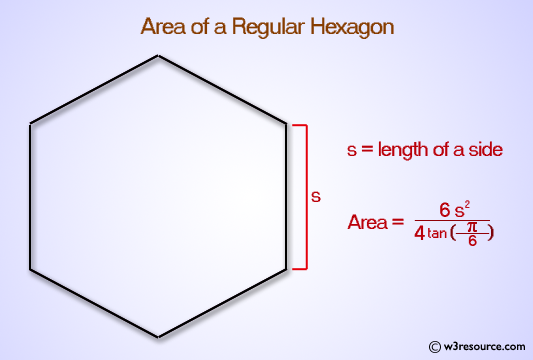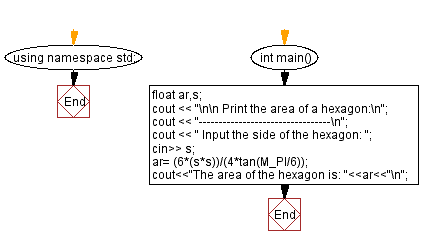﻿ C++ Exercises: Print the area of a hexagon - w3resource

# C++ Exercises: Print the area of a hexagon

## C++ Basic: Exercise-57 with Solution

Write a C++ program to print the area of a hexagon.

Pictorial Presentation:Sample Solution:

C++ Code :

``````#include <iostream>
#include <math.h>
using namespace std;

int main()
{
float ar,s;
cout << "\n\n Print the area of a hexagon:\n";
cout << "---------------------------------\n";
cout << " Input the side of the hexagon: ";
cin>> s;
ar= (6*(s*s))/(4*tan(M_PI/6));
cout<<"The area of the hexagon is: "<<ar<<"\n";

}
``````

Sample Output:

``` Print the area of a hexagon:
---------------------------------
Input the side of the hexagon: 6
The area of the hexagon is: 93.5307
```

Flowchart:C++ Code Editor:

What is the difficulty level of this exercise?

﻿

## C++ Programming: Tips of the Day

Why is there no std::stou?

The most pat answer would be that the C library has no corresponding "strtou", and the C++11 string functions are all just thinly veiled wrappers around the C library functions: The std::sto* functions mirror strto*, and the std::to_string functions use sprintf.

Ref: https://bit.ly/3wtz2qA

We are closing our Disqus commenting system for some maintenanace issues. You may write to us at reach[at]yahoo[dot]com or visit us at Facebook